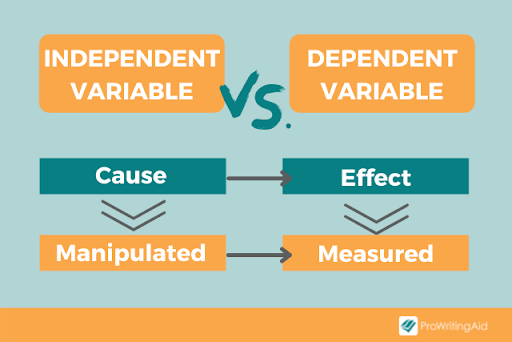# Independent Variable

Definition of Independent Variable:

« Back to Glossary Index

One of the core concepts of Six Sigma is the formula, Y=f(X). In words, this says Y is a function of X. Y is thought of as the dependent variable and X as the independent variable. Let’s explore this concept in more detail and understand why it is important.

## Overview: What is an independent variable?

In the introduction above, you learned that Y=f(X) says that Y is a function of X. If you restate this another way, you can say that Y is dependent on the independent variable, X. Therefore, you can call Y the dependent variable and X the independent variable. Y is independent because it can take on any value and is independent of Y. Since the value of Y will depend on the value of Y, you can refer to Y as the dependent variable.

In some cases, the dependent variable is referred to as the effect while the independent variable is called the cause. In the context of regression analysis the dependent variable is called the predictor variable while the independent variable is called the response variable. In an experiment, you can manipulate the value of the independent variable while the dependent variable will change as a result of your manipulation of the independent variable.

While this may seem confusing, distinguishing between the independent and dependent variables can be accomplished by asking a simple question. As an example, assume you have two variables; height and age. You are interested in which is the independent variable and which is the dependent variable. Ask this question:

Is height dependent upon age or is age dependent on height?

It becomes obvious that your age might cause your height while height does not cause your age. This would make age the independent variable and height your dependent variable.

## An industry example of an independent variable

A Six Sigma Master Black Belt (MBB) recently performed a Design of Experiments (DOE) to determine the effect or response of miles per gallon (mpg) for the company’s truck fleet as a function of multiple factors. The independent variables chosen for the DOE were; speed, gas octane and tire pressure. The MBB set up an experiment to vary the independent variables and then measure the change or response in the dependent variable, mpg. From the results, the MBB was able to determine which independent variables were significant and what values of them would optimize the mpg.

## Frequently Asked Questions (FAQ) about an independent variable

### 1. What is the difference between a dependent and independent variable?

The graphic below presents a clear explanation of the difference.Dependent vs Independent Variable

### 2. What common Six Sigma tools use the concept of independent variable?

One common tool is the Cause and Effect or Fishbone Diagram. The dependent variable would be the Effect or head of the fish while the multiple independent variables would be the Cause or bones of the fish. The other common tool is regression analysis where the response variable is the dependent variable and the predictor variables are the independent variables.

### 3. Can I change the value of an independent variable?

Yes, that is the purpose of such tools as Design of Experiments (DOE). The purpose of DOE is to change the values of the independent variables and observe what happens to the response or dependent variable.

« Back to Dictionary Index